# Olympiad Test: Linear Equations In One Variable - 2

## 10 Questions MCQ Test Mathematics Olympiad Class 7 | Olympiad Test: Linear Equations In One Variable - 2

Description
Attempt Olympiad Test: Linear Equations In One Variable - 2 | 10 questions in 20 minutes | Mock test for Class 7 preparation | Free important questions MCQ to study Mathematics Olympiad Class 7 for Class 7 Exam | Download free PDF with solutions
QUESTION: 1

### The sum of two consecutive even number is 98. What is the smaller number?

Solution:

Let the two consecutive even number be x and x + 2.
∴ x + x +2 = 98
⇒ 2x + 2 = 98
⇒ 2x = 98 –2
⇒ 2x = 96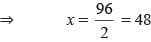QUESTION: 2

### On adding nine to the twice of a whole number gives 61. What is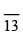of that whole number?

Solution:

Let the whole number be x.
∴ 2x + 9 = 61
⇒ 2x = 61–9
⇒ 2x = 52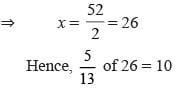QUESTION: 3

### After 12 years Manish will be three times as old as he was 4 years ago. What is his present age?

Solution:

Let the present age be x years.
∴ 4 years ago, his age = (x – 4) years.
After 12 years, his age = (x + 12) years.
Hence, x  + 12 = 3(x – 4)
⇒ x + 12 = 3x –12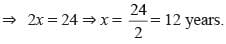QUESTION: 4

Niraj is 19 years younger than his cousin after 5 years their age will be in the ratio 2:3. What is the present age of Niraj?

Solution:

Let the present age of Niraj be x years.
His cousin’s age = x + 19
After 5 years,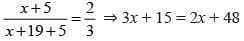⇒ 3x – 2x = 48 –15
⇒ x = 33

QUESTION: 5

What is the value of p in the given equation 8(2p – 5) – 6(3p – 7) = 1?

Solution:

Given 8(2p – 5) – 6(3p – 7) = 1
⇒ 16p – 40 – 18p + 42 = 1
⇒ –2p + 2 = 1
⇒ –2p = 1– 2
⇒ –2p = –1
⇒ p = 1/2

QUESTION: 6

What is the value of y in the given equation 0.6 y  + 0.8 = 0.56y + 2.32?

Solution:

Given equation is
⇒ 0.6 y  + 0.8 = 0.56 y + 2.32
⇒ 0.6 y –  0.56 y = 2.32 – 0.8
⇒ 0.04 y = 1.52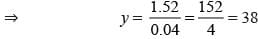QUESTION: 7

Two complementary angles are differ by 14°. What is the measure of larger angle?

Solution:

Let one angle be x.
∴ Other angle = x + 7
Now x  + x + 14 = 90°
⇒ 2x = 90 – 14
⇒ 2x = 76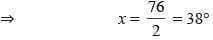QUESTION: 8

The sum of three consecutive odd numbers is 147. What is the smallest odd number?

Solution:

Let the odd numbers are x, x + 2, x + 4.
∴ x + x + 2 + x  + 4 = 147
⇒ 3x + 6 = 147
⇒ 3x = 147 – 6
⇒  3x = 141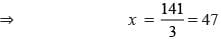Hence, the numbers are 47, 49, 51
∴ Smallest number = 47.

QUESTION: 9

In an isosceles triangle, the vertex angle is thrice of its base angle. What is the measure of vertex angle?

Solution:

Let the base angle be x
Vertex angle = 3x.
∴ x + x + 3x = 180°
⇒ 5x = 180°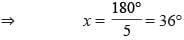Vertex angle = 3×36° = 108°

QUESTION: 10

A number is 2/5 times of another number. If their sum is 140, find the larger number.

Solution: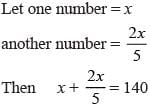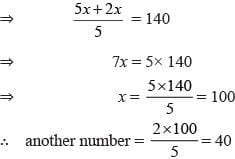Use Code STAYHOME200 and get INR 200 additional OFF Use Coupon Code